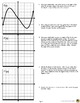# Graphing the Derivative of a Function: Inquiry ActivitySubject
Resource Type
File Type

PDF

Product Description
Graphs of Derivatives - Discovery:

This three-page worksheet will guide your students to graph the derivative of a function and make observations about the following concepts:

* The slope of a tangent line to a curve can be identified at various points and used to create the graph of the derivative.
* The degree of f’(x) is equal to the degree of f(x) minus one.
* The second derivative of f(x) is denoted by f’’(x) and is the derivative of f’(x).
*The derivative of a cubic function is a quadratic function. The derivative of a linear function is a constant…etc.

Students can complete this activity even if they cannot yet differentiate. They must have some prior knowledge of parent functions and be familiar with the term "degree" of a function.

The questions are set up as a guided lab and students make discoveries in an inquiry style. They must answer critical thinking questions in complete sentences.

Students will need a straightedge.

Check out these other resources as well:
Derivative Infographic
Factoring Polynomials "GridWords" : FULL SET
Systems of Equations: "Choose Your Own Journey" book

Total Pages
Included
Teaching Duration
40 minutes
Report this Resource to TpT
Reported resources will be reviewed by our team. Report this resource to let us know if this resource violates TpT’s content guidelines.